Latest Banking jobs   »   Basic Quantitative Aptitude Quiz for All...

# Basic Quantitative Aptitude Quiz for All Banking Exams- 11th May

Q1. Compound interest and simple interest on a certain sum for two years are Rs. 1100 and Rs. 1000 respectively. If rate of interest for both S.I. and C.I is 20% p.a., then find the sum.
(a) Rs. 2500
(b) Rs. 2000
(c) Rs.3000
(d) Rs. 1800
(e) Rs. 2200

Q2. Ramesh invests certain sum into two schemes A & B in the ratio of 2 : 3 for 3 years. Scheme A and B offered S.I. at the rate of 10% and 8% per annum respectively. If total S.I. obtained from both schemes after 3 years was Rs. 3300, then find amount invested by Ramesh in scheme A was?
(a) Rs. 5000
(b) Rs. 6000
(c) Rs. 7500
(d) Rs. 8000
(e) Rs. 2500

Q3. In a 64 litre mixture of milk and water the ratio of water to milk is 3 : 5. If x litre water is mixed in this mixture, so the ratio of water to milk becomes 5 : 8. Find the value of x.
(a) 6 l
(b) 4 l
(c) 2 l
(d) 1 l
(e) 5 l

Q4. The ratio of milk and water in a vessel is 5 : 8. If 6 liter of milk added in it ratio of milk to water becomes 7 : 8. Find the initial quantity of mixture in the vessel.
(a) 28 liter
(b) 39 liter
(c) 42 liter
(d) 24 liter
(e) 36 liter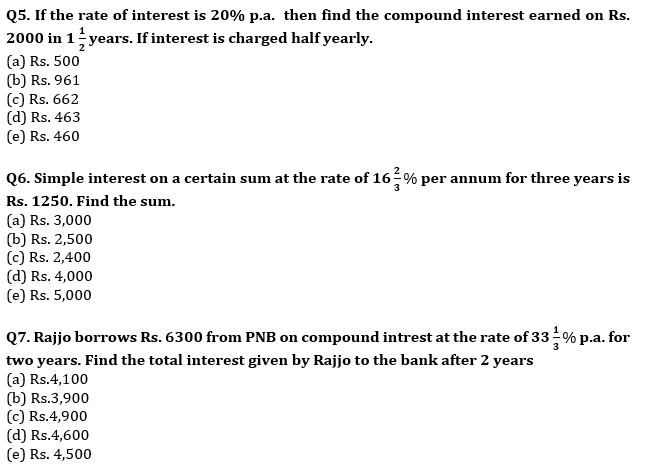Q8. The simple interest on a certain sum for 4 yrs at a rate of 12.5% per annum is Rs 4000. What will be the compound interest on the same sum at a rate of 4% per annum after two years?
(a) Rs 762.8
(b) Rs 562.8
(c) Rs 652.8
(d) Rs 842.4
(e) Rs 648.6

Q9. Bhavya have 10,000 Rs. He invested some amount in Scheme ‘A’ which offers 15% p.a. at SI and rest in Scheme ‘B’ which offers 20% p.a. at CI. Interest earned form scheme ‘A’ after 2 years is 780Rs more than interest earned form scheme ‘B’ after 2 years. Find the amount invested in Scheme ‘B’?
(a) 8000 Rs
(b) 7000 Rs
(c) 3000 Rs
(d) 2000 Rs
(e) 5000 Rs

Q10. A Jar contain water and milk in the ratio of 2: 3. Some milk is added in the Jar whose amount is equal to 30% of water present in the Jar. After this some water is added whose amount is equal to 10% of quantity of milk present in Jar presently. What is the new ratio of water and milk in the Jar.
(a) 59 : 90
(b) 11 : 18
(c) 90 : 59
(d) 18 : 11
(e) 57 : 67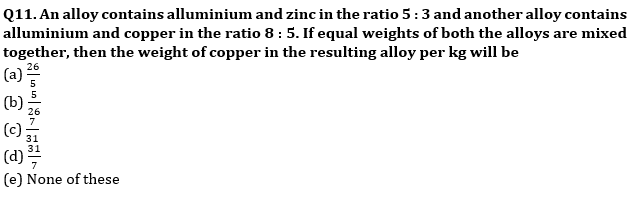Q12. A container carrying wine and water solution in the ratio 7 : 5. 58 litre of water has been added to dilute the solution further more and ratio of the wine and water has been reversed. Find the original volume of the solution?
(a) 130 litre
(b) 244 litre
(c) 248 litre
(d) 145 litre
(e) 184 litre

Q13. A mixture of milk and water contains 75% milk. If 8 liter of mixture is taken out and 7 liters of milk is added, then ratio of milk to water becomes 7 : 2. Find the quantity of mixture initially.
(a) 75 L
(b) 64 L
(c) 68 L
(d) 56 L
(e) 40 L

Q14. In new year offer Axis bank reduced interest rate from 20% to 10% C.I. P.A. Due to this offer Anurag have to pay Rs. 345 less interest in two year. Find the loan amount of Anurag.
(a) Rs. 1,000
(b) Rs. 2,400
(c) Rs. 4,000
(d) Rs. 1,500
(e) Rs. 2,000

Q15. A man invested a certain amount at the rate of 8 % per annum for 5 year and obtained a total SI of Rs. 5000. Had he invested the same amount at the same rate for 2 years, how much amount would he have obtained as CI at the end of 2 year ?
(a) 2050 Rs.
(b) 2010 Rs.
(c) 2040 Rs.
(d) 2080 Rs.
(e) 2040 Rs.

Practice More Questions of Quantitative Aptitude for Competitive Exams:

Solutions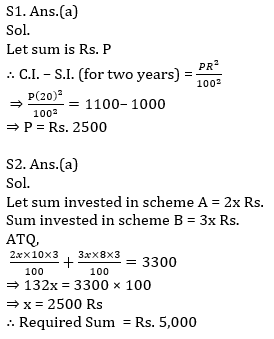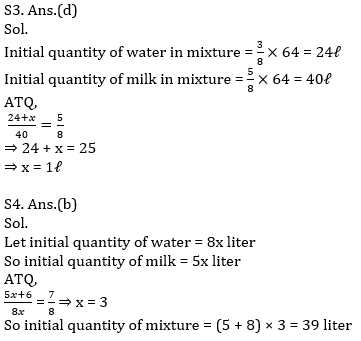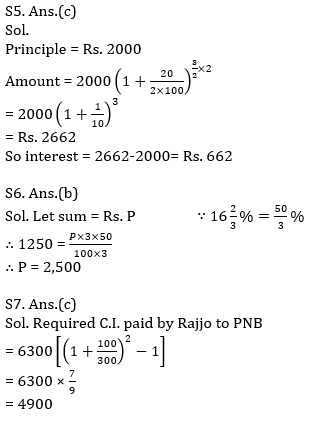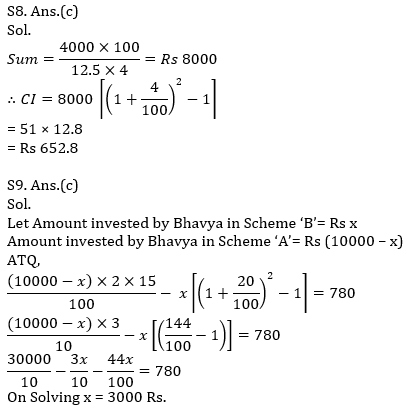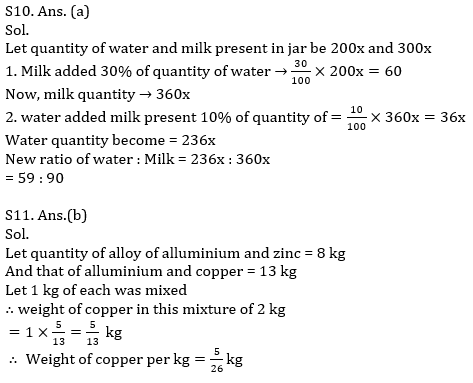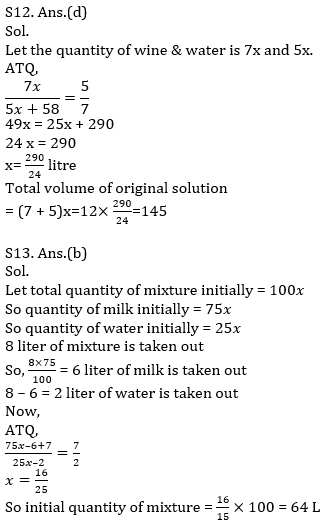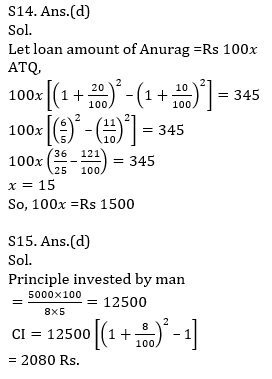#### Congratulations!Incorrect details? Fill the form again here

•Quantitative Aptitude Quiz For IBPS RRB ...
•Quantitative Aptitude Quiz For IBPS Cler...
•Quantitative Aptitude Quiz For IDBI AM/E...
•Quantitative Aptitude Quiz For IDBI AM/E...
•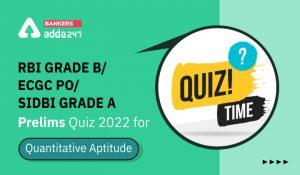Quantitative Aptitude Quiz For RBI Grade...
•Quantitative Aptitude Quiz For SBI Clerk...